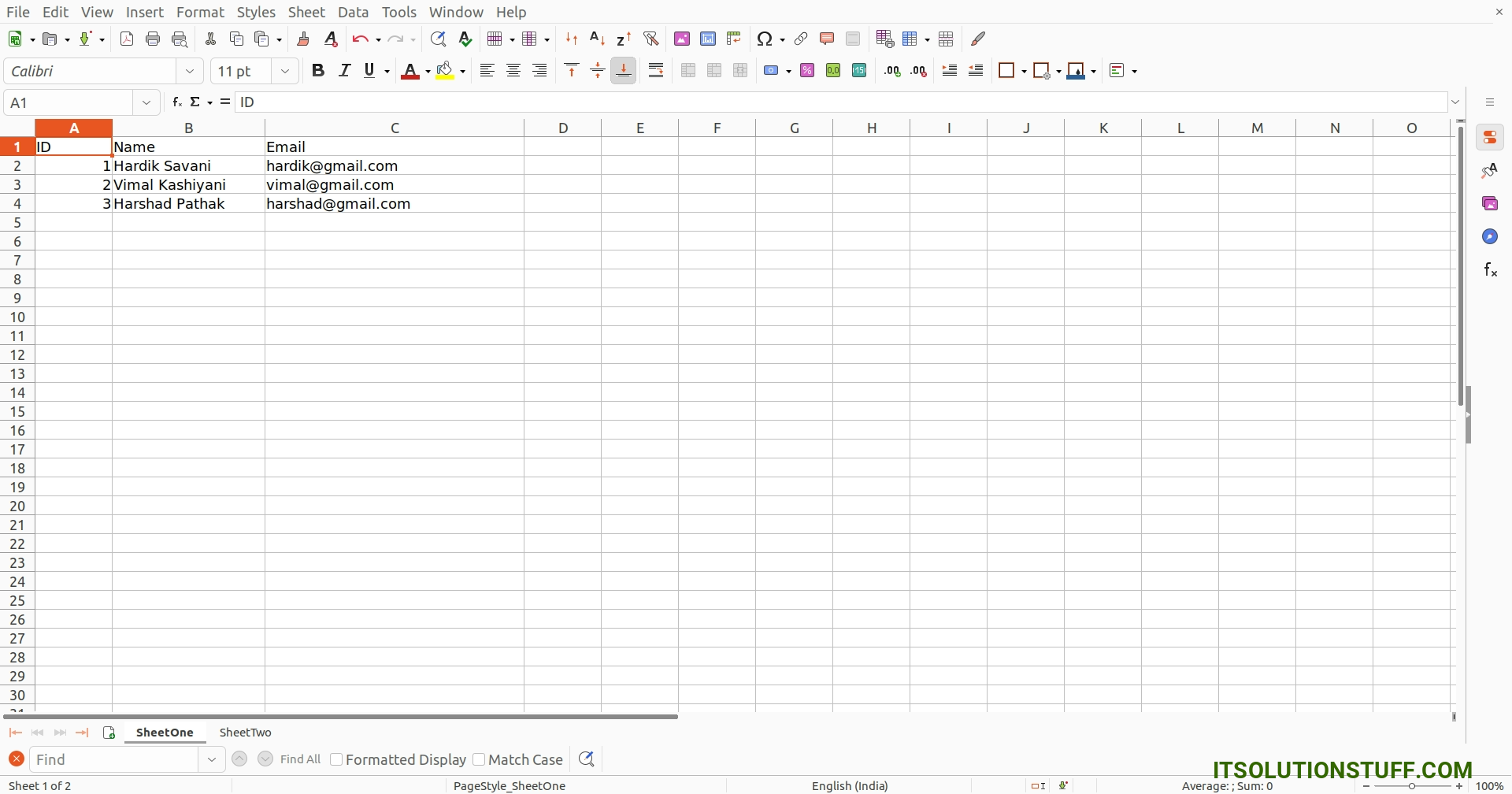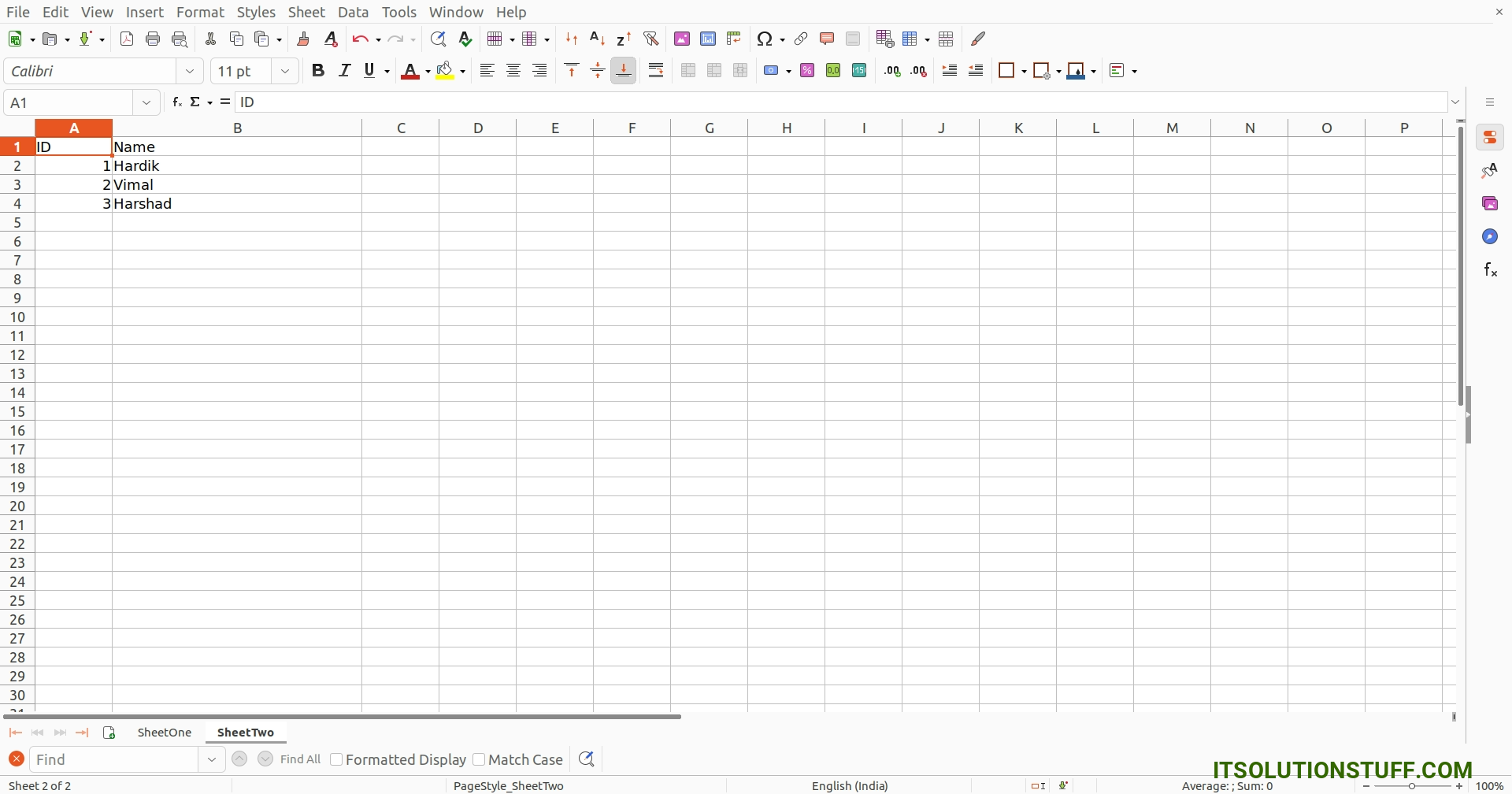# Python Openpyxl Read Excel File Multiple Sheets Example

By Hardik Savani October 30, 2023 Category : Python

Hey Artisan,

In this quick guide, we will teach you python openpyxl read excel multiple sheets. if you want to see an example of python read excel file with multiple sheets openpyxl then you are in the right place. This article will give you a simple example of how to read multiple sheets in excel using python openpyxl. you'll learn python openpyxl read xlsx multiple sheets. Alright, let us dive into the details.

In this example, we will read excel file with multiple sheets using python openpyxl library. we will use load_workbook() and range() functions to read excel file with multiple sheets using python openpyxl. so let's see a simple example.

You can use these examples with python3 (Python 3) version.

I simply created data.xlsx file with SheetOne and SheetTwo as like below, we will use same file for both example:

demo.xlsx: SheetOnedemo.xlsx: SheetTwoExample:

main.py

```import openpyxl

# Define variable to load the dataframe

sheetOne = dataframe['SheetOne']

# Iterate the loop to read the cell values
for row in range(0, sheetOne.max_row):
for col in sheetOne.iter_cols(1, sheetOne.max_column):
print(col[row].value)

sheetTwo = dataframe['SheetTwo']

# Iterate the loop to read the cell values
for row in range(0, sheetTwo.max_row):
for col in sheetTwo.iter_cols(1, sheetTwo.max_column):
print(col[row].value)
```

Output:

```ID
Name
Email
1
Hardik Savani
hardik@gmail.com
2
Vimal Kashiyani
vimal@gmail.com
3

ID
Name
1
Hardik
2
Vimal
3